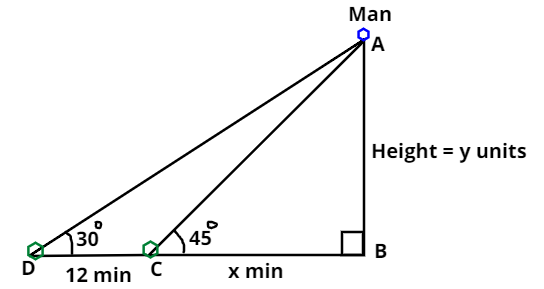Courses
Courses for Kids
Free study material
Free LIVE classes
MoreLIVE
Join Vedantu’s FREE Mastercalss

# A man on the top of a vertical observation tower observes a car moving at auniform speed coming directly towards it. If it takes 12 minutes for the angle ofdepression to change from ${30^0}$ to ${45^0}$. How soon after this will the car reach theobservation tower?$(A){\text{ 14min 3 sec}} \\ (B){\text{ 15min 49sec}} \\ (C){\text{ 16min 23sec}} \\ (D){\text{ 18min 5sec}} \\$Verified
363.6k+ views
Hint: Draw figure and then use trigonometry identity $\tan \theta = \dfrac{{Perpendicular}}{{Base}}$.Above figure is drawn with respect to the given conditions in question.
As we can see from the above figure that,
Man is on the top of a vertical tower.
And to change angle of depression from ${30^0}$ to ${45^0}$ i.e. $\angle {\text{ADB}}$ to $\angle {\text{ACB}}$.
It takes 12 minutes,
And it is obvious that when the car will reach the observation tower,
then the angle of depression will be ${90^0}$.
Let the height of the tower be $y$ units.
As we are given that the time taken to travel DC (see in figure) is 12 minutes.
Let the time taken to travel CB will be $x$ minutes.
Here we are known with perpendicular and base of $\Delta {\text{ABC}}$ and $\Delta {\text{ABD}}$.
So, we will only use that trigonometric functions, that include perpendicular and base
So, as we know that, $\tan \theta = \dfrac{{Perpendicular}}{{Base}}$.
So, as we can see from the above figure, $\tan {45^0} = \dfrac{{AB}}{{CB}} = \dfrac{y}{x}$.
So, $x = y$ ……………………………………….(1)
And, $\tan 30^\circ = \dfrac{{AB}}{{DB}} = \dfrac{{AB}}{{DC + CB}} = \dfrac{y}{{12 + x}}$.
Now, putting the value of $\tan {30^0}$ and $y$ from equation 1. We get,
$\dfrac{1}{{\sqrt 3 }} = \dfrac{x}{{12 + x}} \Rightarrow \left( {\sqrt 3 - 1} \right)x = 12 \Rightarrow x = \dfrac{{12}}{{\left( {\sqrt 3 - 1} \right)}} \approx 16.38$minutes
Now, as we have defined above that time taken to travel CB is x minutes.
So, time taken to reach the observation tower will be x minutes.
So, according to the options given in the question
the most appropriate answer will be 16min 23 sec.
Hence, the correct Option will be C.

Note: Whenever we come up with these types of problems first, we should draw a figure according to the given conditions in question. And then we will assume time taken to reach the tower as x and then after using trigonometric functions like ${\text{tan}}\theta$, we can get the value of x using angle of depression and time taken to change angle of depression. This will be the easiest and efficient way to reach the required solution of the problem.
Last updated date: 23rd Sep 2023
Total views: 363.6k
Views today: 10.63k